Algebra 1 1-6 Complete Lesson: Multiplying and Dividing Real Numbers
starstarstarstarstarstarstarstarstarstar
5 (1 rating)
by Matthew Richardson
| 30 Questions
Note from the author:
A complete formative lesson with embedded slideshow, mini lecture screencasts, checks for understanding, practice items, mixed review, and reflection. I create these assignments to supplement each lesson of Pearson's Common Core Edition Algebra 1, Algebra 2, and Geometry courses. See also mathquest.net and twitter.com/mathquestEDU.The outlined content above was added from outside of Formative.1
2
1
10 pts
Solve It! What is the sign of the product of a positive number and a negative number?
Positive
Negative
2
10 pts
Solve It! What is the sign of the product of two negative numbers?
Positive
Negative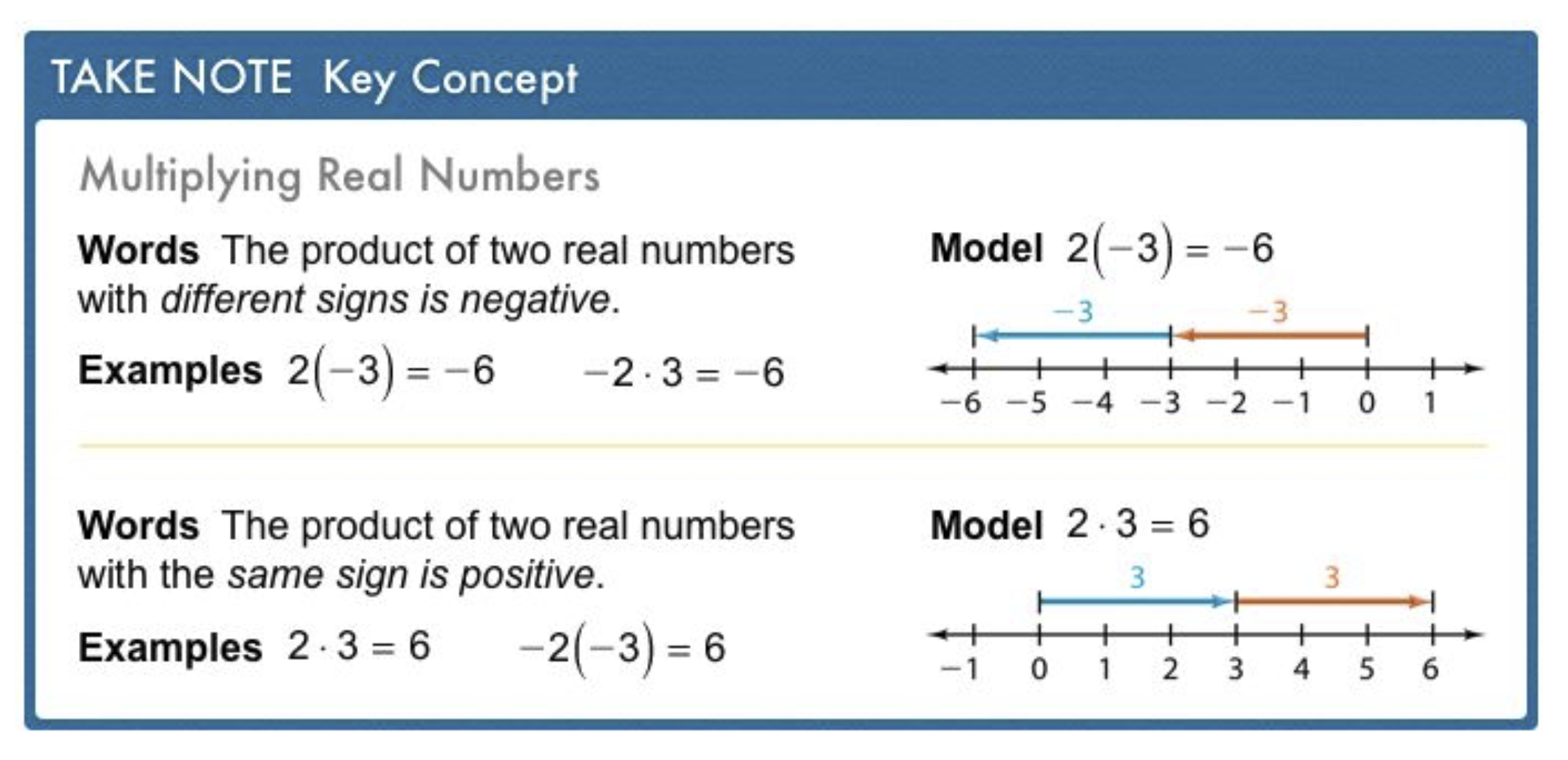3
3
10 pts
Problem 1 Got It?
A
B
C
D4
4
10 pts
Problem 1 Got It?
A
B
C
D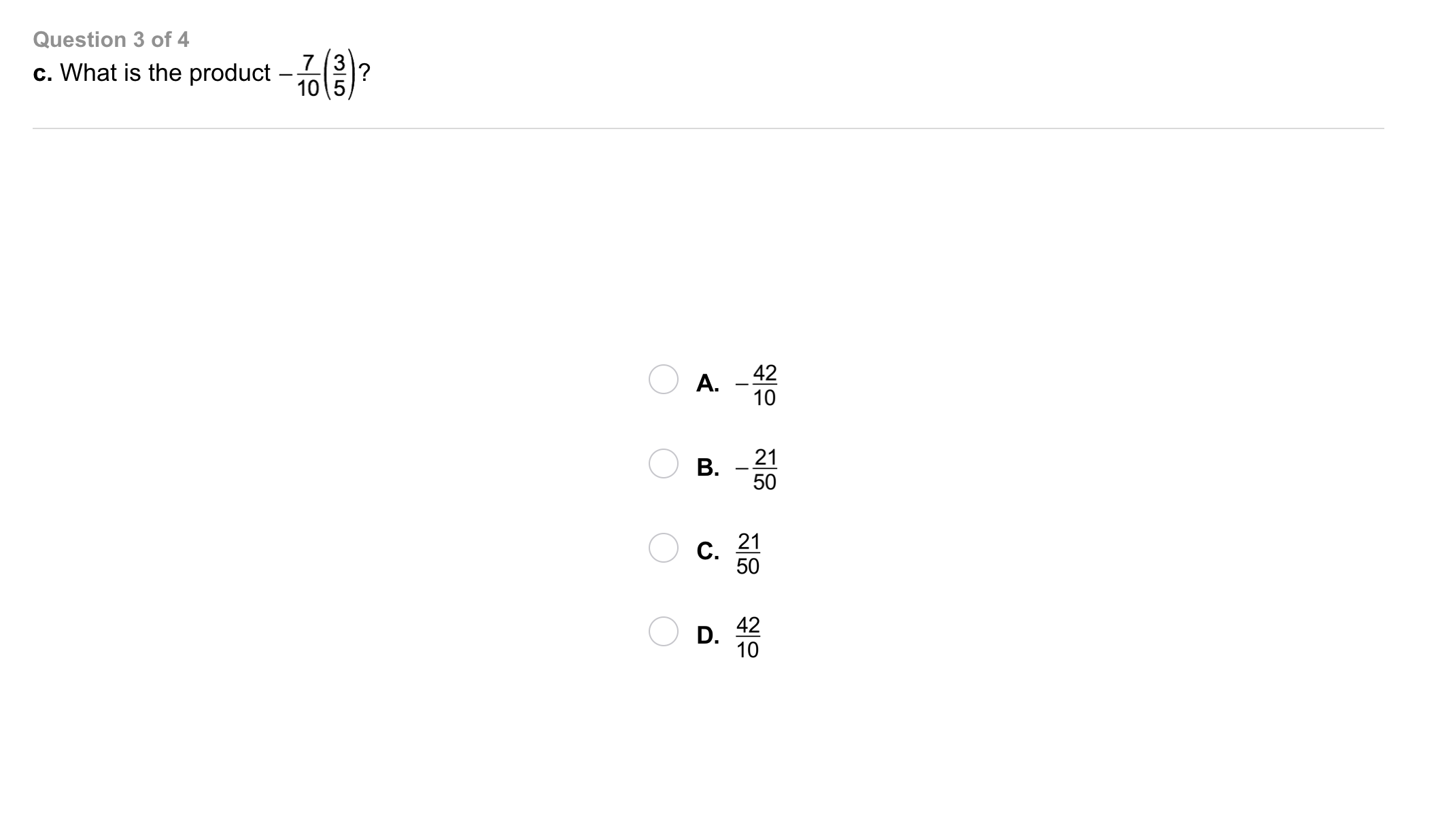5
5
10 pts
Problem 1 Got It?
A
B
C
D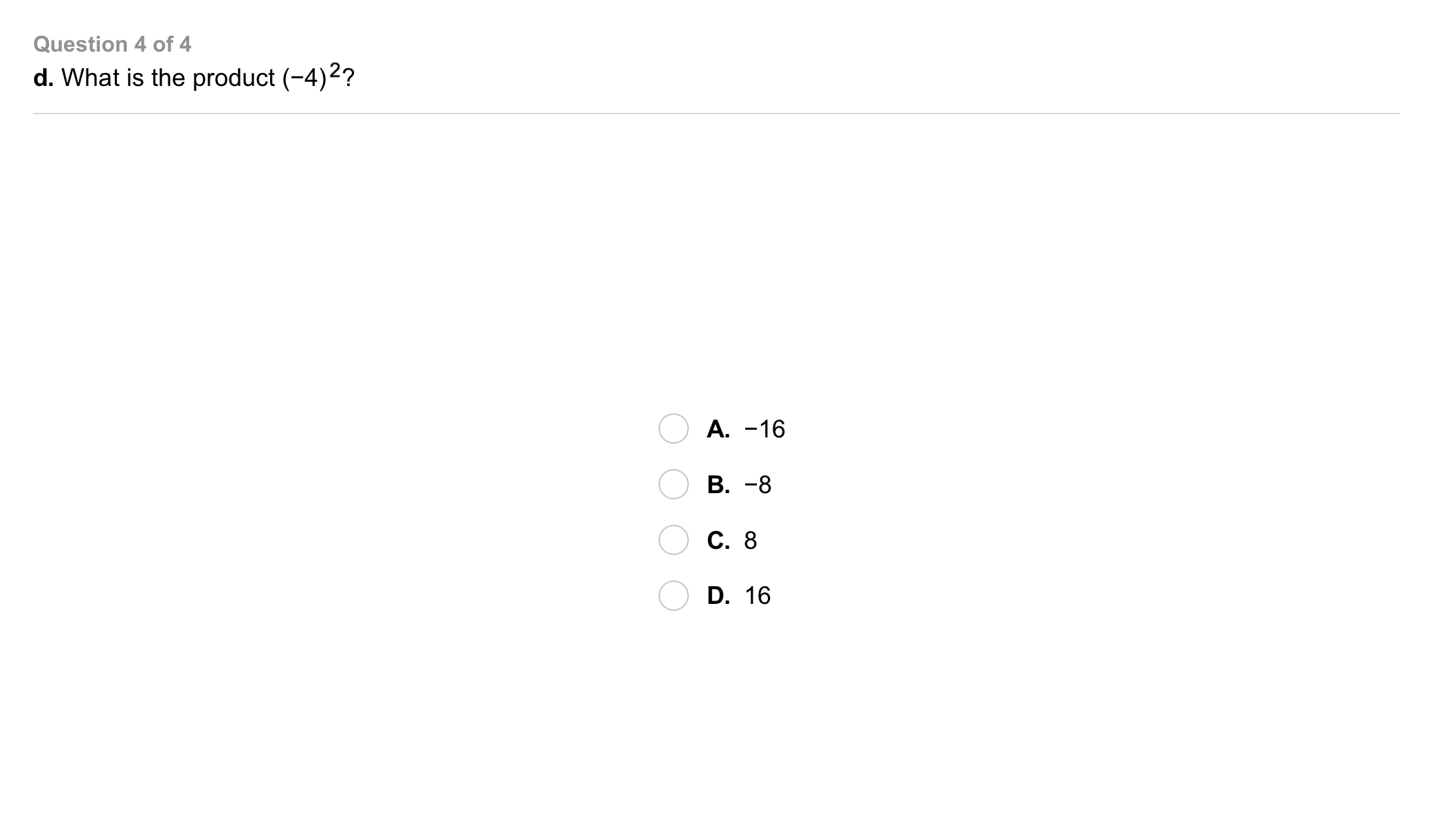6
6
10 pts
Problem 1 Got It?
A
B
C
D7
7
10 pts
Problem 2 Got It?
A
B
C
D8
8
10 pts
Problem 2 Got It?
A
B
C
D9
9
10 pts
Problem 2 Got It?
A
B
C
D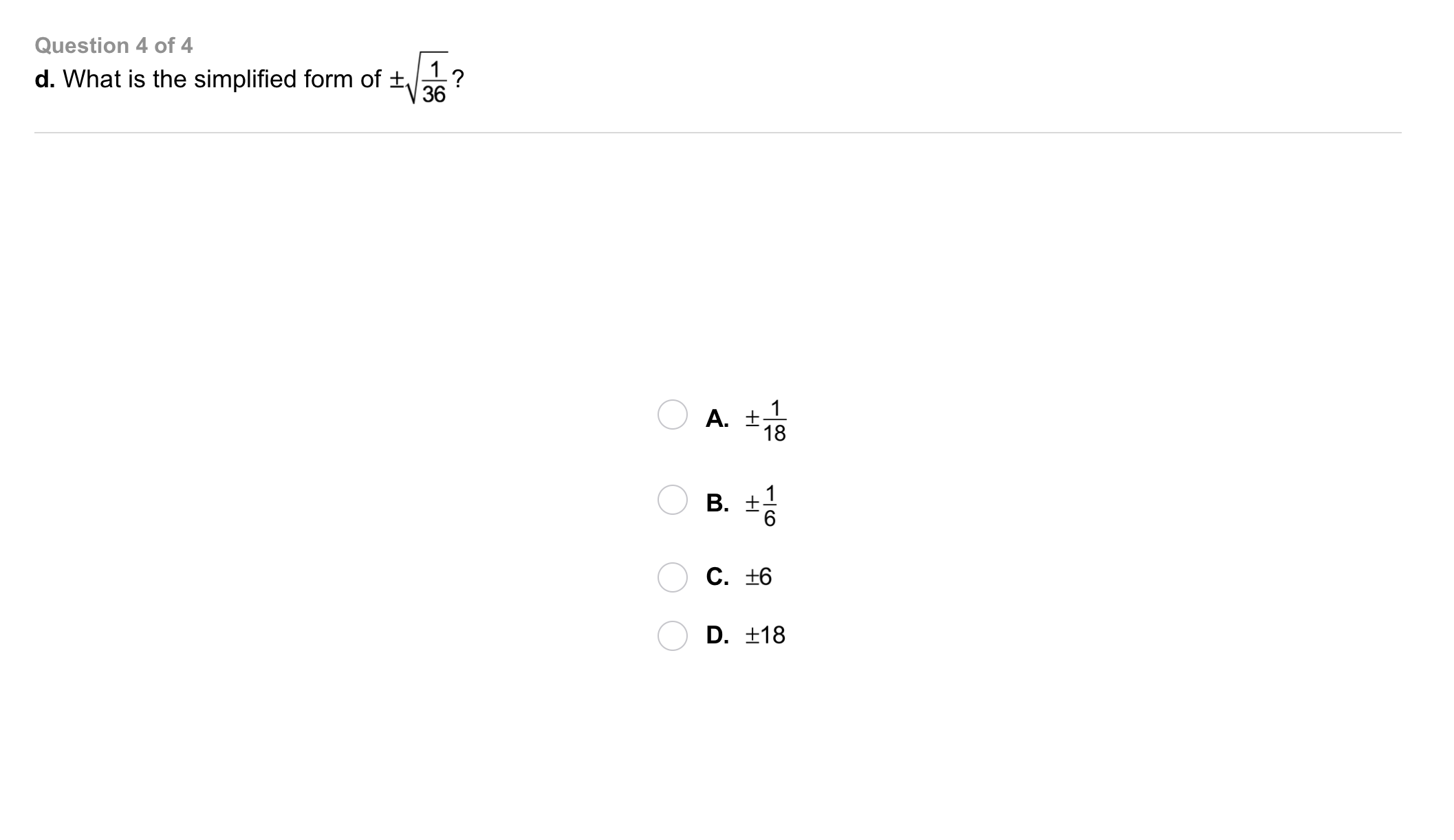10
10
10 pts
Problem 2 Got It?
A
B
C
D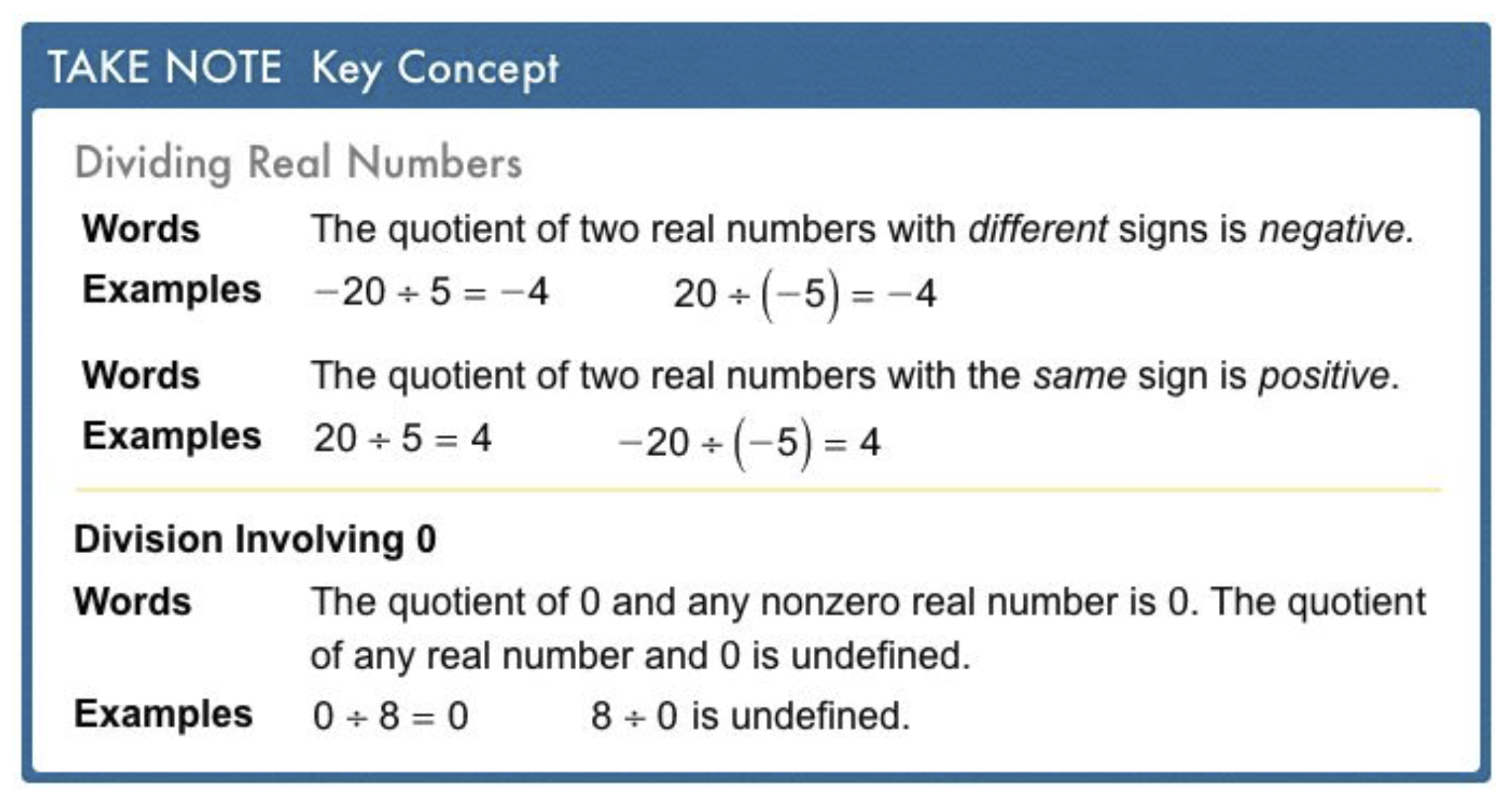11
11
10 pts
Problem 3 Got It?
A
B
C
D12
10 pts
Problem 4 Got It? What is the value of the expression?
Write only a number, in fraction format.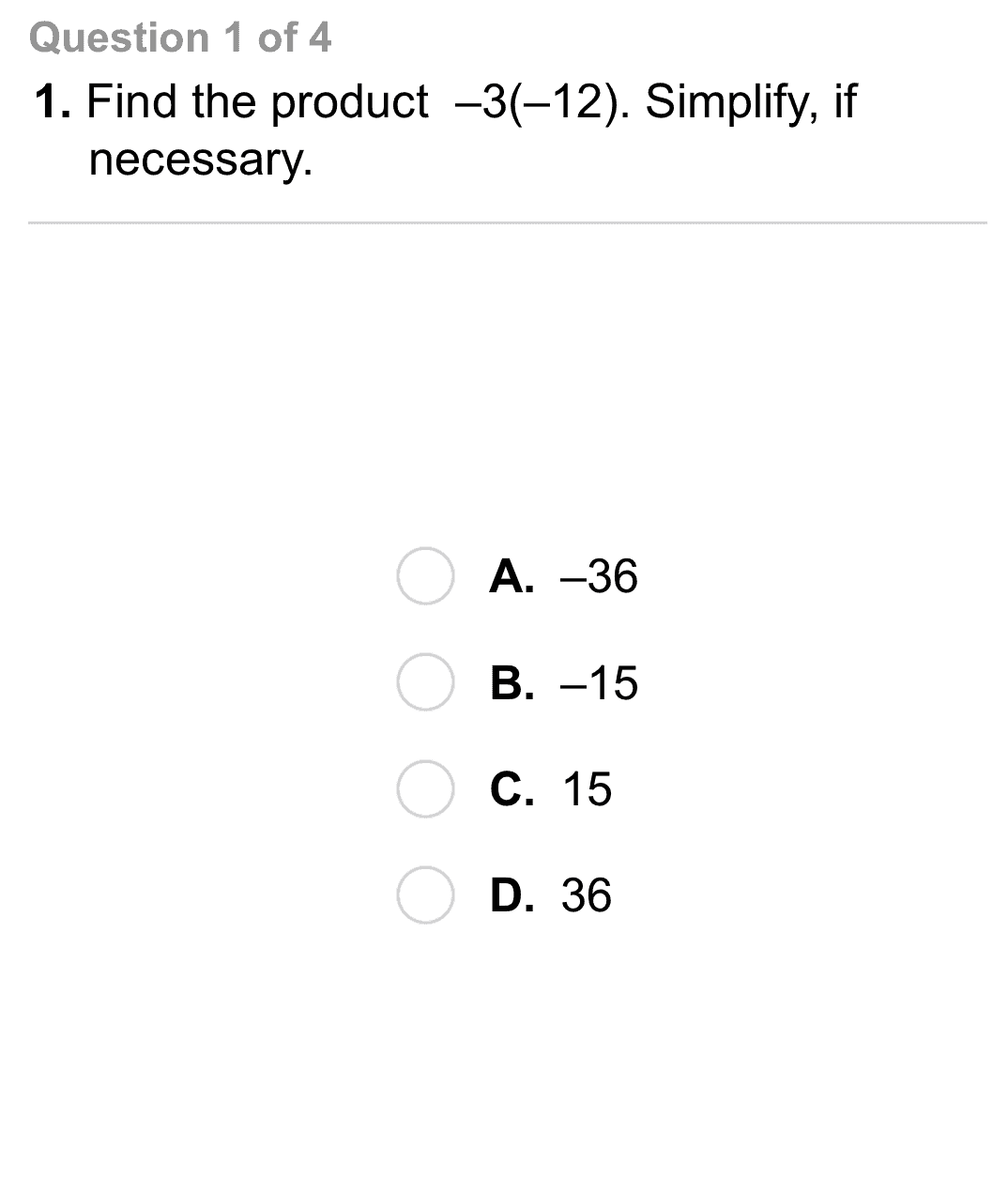14
14
10 pts
A
B
C
D15
15
10 pts
A
B
C
D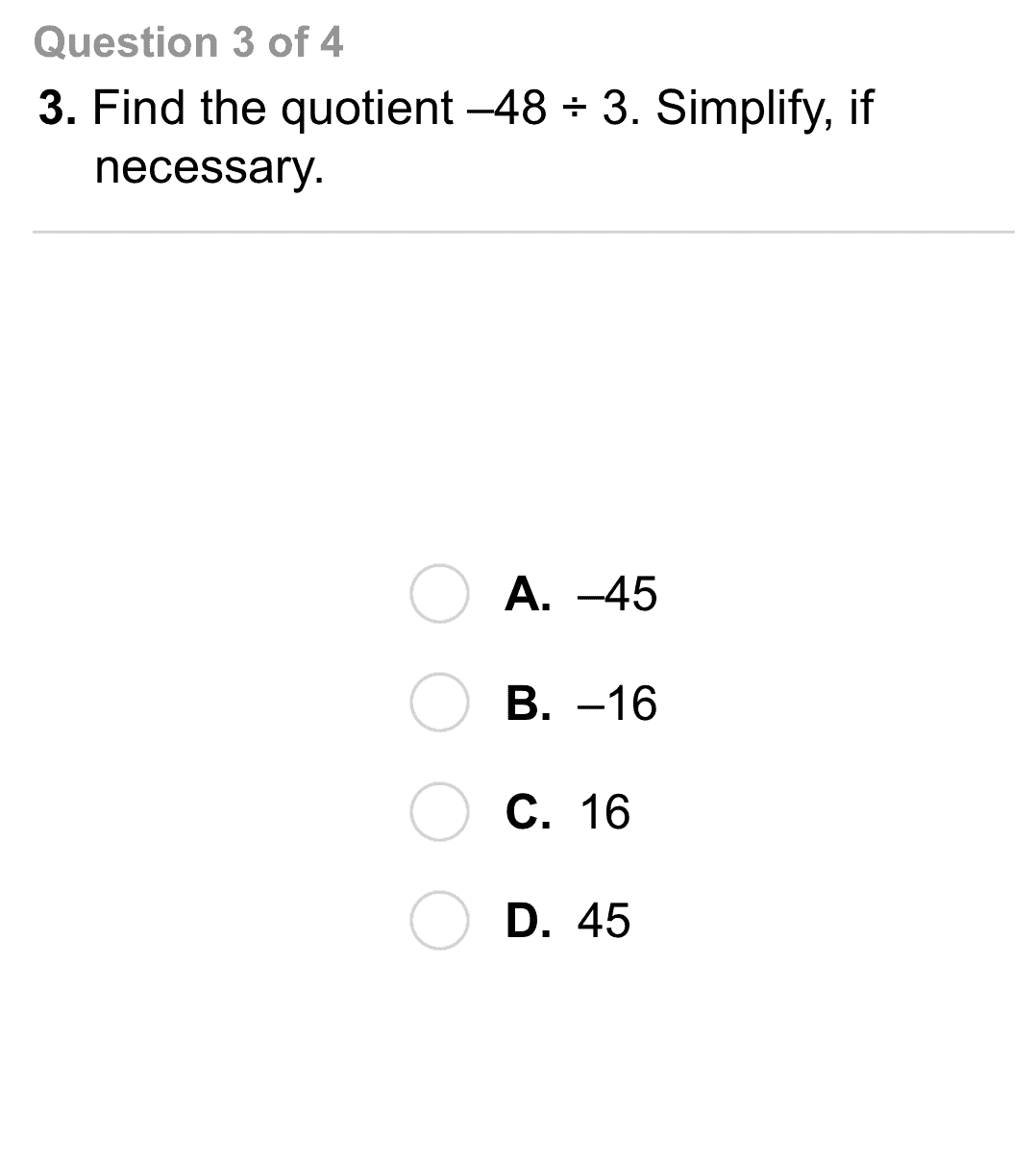16
16
10 pts
A
B
C
D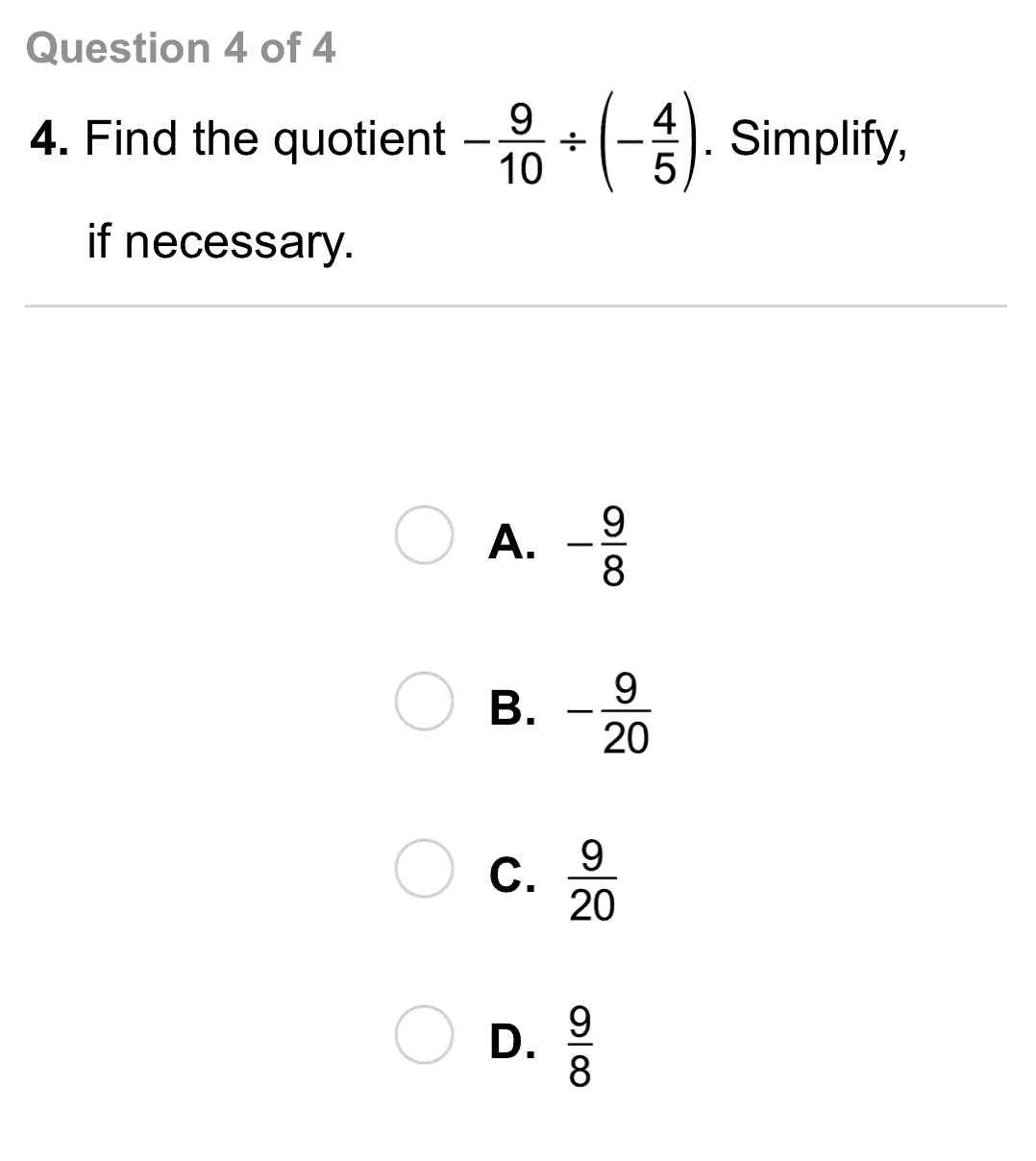17
17
10 pts
A
B
C
D18
10 pts
Vocabulary: What is the reciprocal of -1/5?

Write only a number in simplest form.
19
10 pts
Reasoning: Use a number line to explain why -15 ÷ 3 = -5.
20
10 pts
Reasoning: Determine how many real square roots 49 has. Explain your answer.
Forty-nine has exactly one square root. Seven times seven equals 49.
Forty-nine has no square roots. No integer times itself equals 49.
Forty-nine has exactly two square roots. Seven times seven AND negative seven times negative seven equals 49.21
5 pts
Review Lesson 1-5: Find the difference.
Enter only a number.
22
5 pts
Review Lesson 1-5: Find the difference.
Enter only a number.
23
5 pts
Review Lesson 1-5: Find the difference.
Enter only a number.
24
10 pts
Review Lesson 1-4: Name the property that the statement illustrates.

Associative property of multiplication
Identity property of subtraction
Zero property of multiplication
Multiplication property of -1
Commutative property of addition
Associative property of addition
Commutative property of multiplication
Identity property of addition
25
10 pts
Review Lesson 1-4: Name the property that the statement illustrates.

Identity property of subtraction
Commutative property of addition
Zero property of multiplication
Commutative property of multiplication
Associative property of addition
Multiplication property of -1
Associative property of multiplication
Identity property of addition
26
10 pts
Review Lesson 1-4: Name the property that the statement illustrates.

Multiplication property of -1
Zero property of multiplication
Identity property of subtraction
Associative property of addition
Identity property of addition
Commutative property of multiplication
Commutative property of addition
Associative property of multiplication27
10 pts
Vocabulary: Identify the product and quotient of 12 and 4.
• 3
• 8
• 16
• 48
• Product of 12 and 4
• Quotient of 12 and 428
10 pts
Use Your Vocabulary: Match each number on the left with its reciprocal on the right.

29
100 pts
Notes: Take a clear picture or screenshot of your Cornell notes for this lesson. Upload it to the canvas. Zoom and pan as needed.

For a refresher on the Cornell note-taking system, click here.
30
10 pts
Reflection: Math Success
Add to my formatives list

Formative uses cookies to allow us to better understand how the site is used. By continuing to use this site, you consent to the Terms of Service and Privacy Policy.